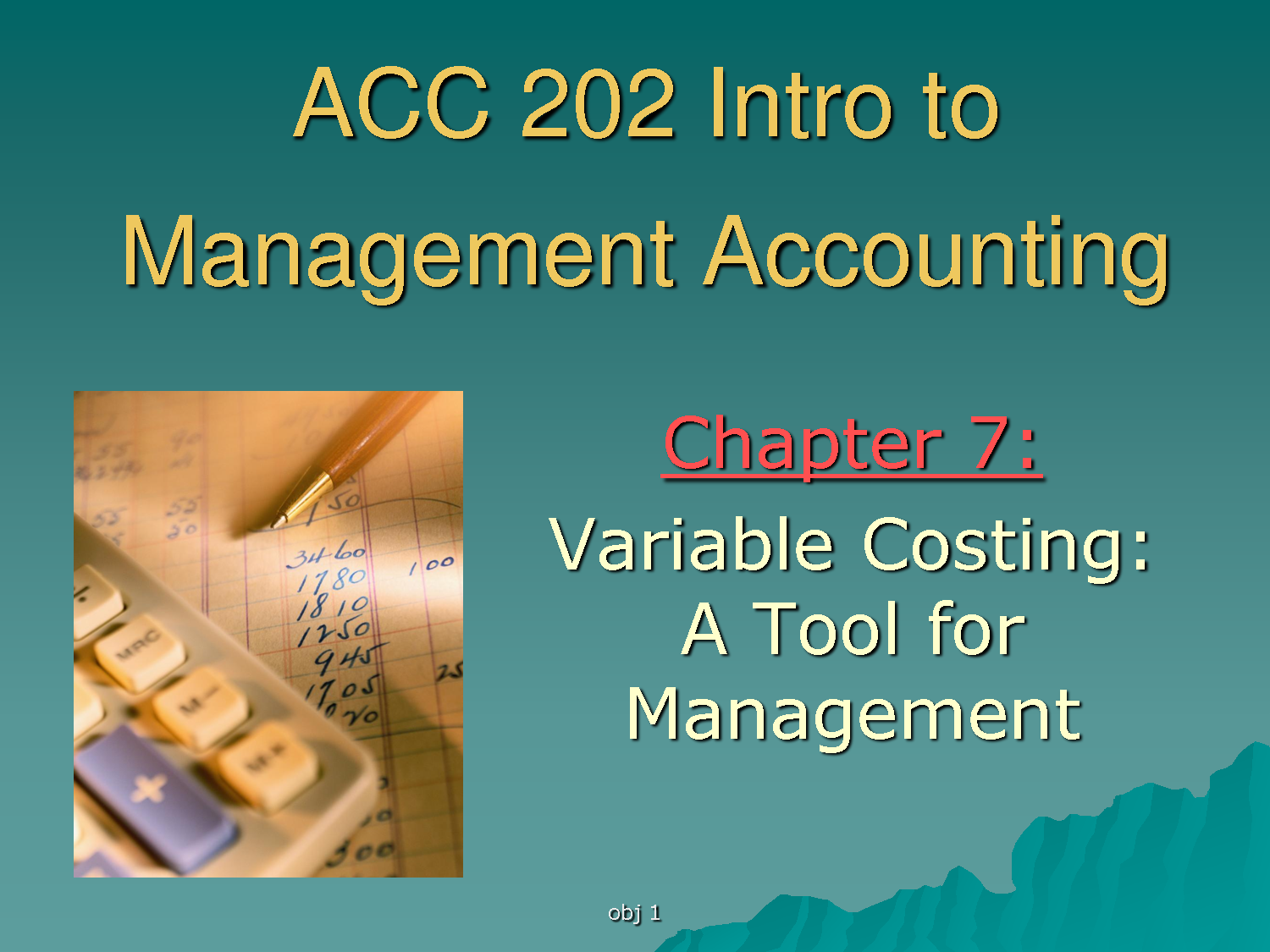Accounting

# Lecture on Variable CostingVariable costs are expenses that change in proportion to the activity of a business. Variable costing is the sum of marginal costs  over all units produced. It can also be considered normal costs. Fixed costs and variable costs make up the two components of total cost. Direct Costs, however, are costs that can easily be associated with a particular cost object. However, not all variable costs are direct costs. Variable costs are sometimes called unit-level costs as they vary with the number of units produced.